American Journal of Physics and Applications
Volume 3, Issue 3, May 2015, Pages: 69-76

Einstein: Unification Theory and Unification of Basic Forces

M. Tajuddin1, Md. Haider Ali Biswas2

1Directorate of Primary Education, Ministry of Pry. Education, Dhaka, Bangladesh

2Mathematics Discipline, Khulna University, Khulna, Bangladesh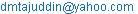(M. Tajuddin)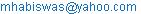(Md. H. A. Biswas)

M. Tajuddin, Md. Haider Ali Biswas. Einstein: Unification Theory and Unification of Basic Forces. American Journal of Physics and Applications. Vol. 3, No. 3, 2015, pp. 69-76. doi: 10.11648/j.ajpa.20150303.12

Abstract: This is a unique article that expresses the idea of unification theory and unification of basic forces. Depending on the truth that ‘every particle feels the force of gravity according to its mass or energy’ (Hawking, 1996) and spherical N-dimension of geometry by Riemann, it is observed how gravitation as a long range virtual particle spherically interacts with short range forces lying in inertia of mass in the earth and the other planets and form unified force to control the universe with spherical motion. Without mutual interaction of the four basic forces, gravitation and unified force is impossible. Unified force creates spherical geometry that incorporates two dimensional, three dimensional, four dimensional and curved geometry by single dimension. As ‘unified theory that would include all these partial theories (Hawking, 1996), so it can describe all physical laws. The basic forces are virtual but the reactions are visible as the planets have spherical movement etc. (Vide: Different sections of the article).

Keywords: Unification Theory, Unified Force, Spherical Motion, N-Dimensions, Single Dimension

1. Introduction

Newton formulated law of gravitation in 1687 and afterward people began to think that gravitation controls the universe. But this article expresses that gravitation alone cannot control the universe without the help of unified force and we may call this unified force is also a great force. Though quantum mechanics derives from theoretical physics but it has its own domain, thus unification theory is proved here by both the theories that one cannot refuse the other. Einstein first initiated unification theory in theoretical physics and afterward in quantum mechanics. In theoretical physics ‘Einstein wished to relate all the properties of matter in a unified field theory’(Strathern, 1997). Again Einstein as one of the father of quantum mechanics though differs with Heisenberg, Schrödinger and Dirac in dual principle; he tried to formulate unified force in quantum mechanics. In quantum mechanics ‘Einstein’s main scientific interest was in developing a unified theory, an attempt to explain both gravity and electromagnetism in one mathematical package’-(White and Gribbin, 2005)2. Einstein failed to succeed in both the attempts because we find in Schwarzschild solution that Einstein used Euclidean type of zero tensors in his metric (vide: Einstein’s law of gravitation).

It is need less to write the definition and importance of unification theory because physicists want to know how the universe is working by the unified force. We may call unified force is a great force though it is created by four basic forces of quantum mechanics and some properties of matter of theoretical physics. The two systems are interdependent in quest of unification theory. The main aspect of the study of unified force is to know the techniques it uses to control the universe and how to utilize these techniques for the wellbeing of mankind. To fulfill the genius of two categories of physicists like theoretical and quantum, unification theory is solved here keeping in view both sides. Matter has some inbuilt quality known as property. No doubt, basic forces are properties of matter. At first four basic forces of quantum mechanics such as gravitation, electromagnetic, weak and strong nuclear forces form unified force and in theoretical physics these four properties of matter also creates unified force and in both the cases some extra properties such as radius of circle, cyclic frequency, mass of the body and time join unified force to make it workable. This theory is explained here two ways: first, how unification theory is solved by quantum mechanics as well as by theoretical physics interdependently. Secondly, what is the trend of physics and mathematics from ancient Greek to the present days and how far this progress favors or disfavors unification theory.

2. Unified Force

Unification theory is expressed here by both the theories interdependently that one group may not refuse the other. It is possible because both the theories meet in the elementary level. ‘Einstein wished to relate all the properties of matter in a unified field theory’ (Strathern, 1997). Einstein found that space-time is curved and gravitation is also curved and they curve all motions. But he could not succeed in his attempts. According to Einstein’s equation E= mc2 inertial mass of the earth equals to total energy of the earth. In theoretical physics, properties of matter include four basic forces of quantum mechanics. The earth is a big inertial mass as well as a solid sphere. The earth with all properties of matter and basic forces moves around the sun. Again in quantum mechanics ‘Einstein’s main scientific interest was in developing a unified theory, an attempt to explain both gravity and electromagnetism in one mathematical package’ (White and Gribbin, 2005). But Einstein could not succeed in both the attempts because we know from Schwarzschild solution that he used Euclidean type of zero tensors in his metric.

The amount of solar energy discharges by the sun is1.35´103watt/m2. As a result the mass of the sun decreases 4.5´106tons per second. Where does go the solar energy? The sun has axial and orbital movements and as a result the long range virtual particles discharged from the sun reach the earth spherically to its three basic short range forces such as electromagnetic, weak and strong nuclear forces lying in the inertia mass of the earth. ‘Every particle feels the force of gravity according to its mass or energy’ (Hawking, 1996). So the long range forces have appeal to the short range forces in inertia mass of the earth. The long range virtual particles discharging from the sun induce to the three basic forces of inertia of mass of the earth and the short range forces sustain that force and thus results in unified force. The long range particles spherically interact with the short range three basic forces lying in the inertia of mass of the earth. The earth with its vast inertial mass rotates on its own axis and also around the sun due to spherical attraction of unified force. This unified force controls the universe. At first, unified force is formed by four basic forces as well as by the same four properties of matter (vide: Structure of unified force). In a nutshell, four types of piloting are necessary to control the earth and the planets and inertia of mass such as one pilot remain outside the earth and other three inside. It reminds us how Apollo 11 in 1969 conquered the moon where Collins remained outside the moon in the spacecraft and Armstrong and Aldrin landed on the moon. It is stated here how both the theories form unified force in elementary level.

3. Where is the Problem

The Greek mathematician Euclid developed geometry considering the earth as flat and static and his geometry was the foundation of plane geometry. But the earth is spherical and it has cyclic movement. ‘Thus Euclidean geometry was no longer sufficient to describe the universe’ (Strathern, 1997). But Euclidean geometry is still used for practical purpose. ‘Newton followed the model of Euclid’s Elements which had long been a basis for the presentation of mathematical investigation’ (Fauvel, 1988). As a result ‘all the theories of sciences are formulated on the assumption that space-time is smooth and nearly flat’ (Hawking, 1996). As the world is spherical Euclidean geometry can not depict universe properly. Thus it is important to convert the Euclidean geometry into spherical geometry or to devise spherical geometry which is consonance with the spherical motion of the world and by which unification theory is solved.

4. How to Solve the Problem

Galileo first observes that in vacuum all bodies having different mass, if dropped from the same height reach the surface of the earth simultaneously. Galileo shows by dropping stones of different sizes from the Leaning Tower of Pisa and Bologna that they come to the ground at the same time with equal rapidity. Here ‘equal rapidity’ is a deceptive truth because the real truth is hidden behind. Gravitation influences upon all bodies proportionate to the quantities of mass of the bodies directly, not among the bodies themselves. So it is better to use the phrase ‘proportional rapidity’ instead of ‘equal rapidity’. Newton without knowing the real cause of falling bodies he exhibits ‘Guinea and Feather Experiment’. He further deviated from the real truth by developing laws of inertia and law of gravitation separately. The world is a single entity where a single law of motion governs the world. So it was a great violation in physical law(vide: Galileo and His Subsequents ).

Einstein without knowing the cause of falling bodies of Galileo accepted in his principle of equivalence that ‘different masses fall at the same rate’ (Good, 1974). Einstein proceeds further in his principle of equivalence that law of inertia and law of gravitation are equivalent. ‘Experiment has exhibited a remarkable relationship between gravitation and inertia which is expressed in the law of equality of gravitational and inertial mass’ (Born, 1962). In this research it is established that inertia of mass derives from (gravitation) unified force and inertia of mass is not equivalent to gravitational mass and it is a superior to inertia mass. Thus Einstein could not overcome the classical mechanics. ‘This is why the general theory of relativity is called a classical theory’ (Hawking, 1993). One the other hand Einstein observed that space-time is curved and gravitation curves all motions. Due to the spherical interaction of gravitation with three basic forces, all motions become spherical in nature. Thus unification theory establishes unified force and here is the solution. Einstein desired such a unified force in his final effort. In this regard more details can be found in (Tajuddin et al., 2008)

5. Particles Responsible for Unified Force

The four basic forces create unified force by mutual interactions. It may be called fifth force that controls the universe. This force has special features and techniques to handle the universe. ‘In the current classification, two main classes of particles are recognized; leptons (electron, muon and tau particles) which interact either by the electromagnetic interaction or the weak interaction and have no apparent internal structure; and hadrons (nucleons, pions etc.) which interact by the strong interaction and have a complex internal structure’ (Goldstein, 1999). Generally the particles of lepton groups become long range and particles of hadrons groups become short range. It is considered that hadrons group particles remain in inertia of mass and receive the gravitation. We see magnetic induction does not happen without magnetic substance. Gravitation cannot meet inertia of mass unless there are receivers and short range forces are in inertia of masses to receive the gravitation. Recently discovered God particle belongs to lepton groups.

6. Kinds of Properties of Matter

Properties of matter gradually increase its list with the new inventions of quantum mechanics. Matter has some inbuilt nature known as property. Properties of matter include basic forces. These properties are of two kinds as constant and variable. Inertia of mass is of constant properties and other properties of matter such as gravitation, electromagnetic, weak atomic, strong atomic and unified force are variable. The variable properties control the constant property. The earth and the planets are inertial mass of constant property controlled by variable properties of five basic forces.

7. Combination of Unified Force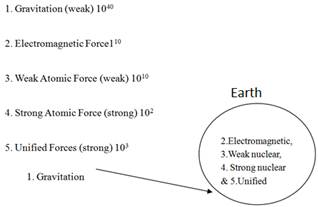Figure 1. Combination of energy in unified forces

The physicists of quantum mechanics have been trying to solve the problem of unification of basic forces for the last one hundred years. ‘Recently, successful attempts have been made to unify three of the four categories of force’ (Hawking, 1996). Gravitation as a long range force interacts with three basic forces that remain inside inertia of mass. When the long range force interacts with three basic forces lying in the inertia, unified force is created. Atom has five layers and every layer contains one type of energy. The layers of atom from upward to inward are as follows: gravitation, electromagnetic, weak, strong nuclear and unified force. Comparison with basic forces electromagnetic force is considered as unit 110. Gravitation is 1040 times and weak atomic force is 1010 times weaker than the electromagnetic force but strong atomic force is 102 times and unified force is 103 times stronger than the electromagnetic force. Thus gravitation is the weakest and unified force is the strongest of the five basic forces (Goldstein, 1999). Four basic forces help the unified force by their energy and they become a sharer of the unified force. The ratio of energy of five basic forces in comparison with electromagnetic force is shown here in Figure 1.

8. Spherical Motion

Gravitation works in spherical way and it is a new addition in unification theory. We are to conceive this though gravitation is a virtual particle. Gravitation has spherical motion otherwise earth would not move around the sun. It is like water cycle, wind cycle, vehicles’ movement cyclic, motor’s movement cyclic, electromagnetic movement cycle etc. Gravitation has own energy as that of solar energy in high space controlling planets. Gravitational cycle may supply energy that may be used in spacecraft and other field as that of solar energy in different fields. Gravitational motion has spherical equation as that of Maxwell’s equations (vide: Spherical motion of Gravitation).

9. Secondly, what is the Trend of Physics

What were the attempts to find out unification theory in previous days? What was the hindrance in the way? This attempt may be in vain unless we analyze the trend of physics and mathematics. It is found that traditional trend of physics and mathematics are somewhat defective and it is a hindrance to find out unification theory.

10. Greek Influence

About two and a half century past a wonderful civilization was flourished in Greece. The Greeks had a wonderful development in different disciplines. ‘Greek had a belief that the earth was stationary and that the sun, the moon, the planets and the stars moved in circular orbit about the earth’ (Hawking, 1996). Greek mathematician Euclid devised Elements considering the earth as flat and static and his geometry subsequently developed is appropriate for two and three dimensional world. In seventeenth century the concept of earth is changed as it is spherical and has axial and orbital movement. ‘Thus Euclidean Geometry was no longer sufficient to describe the universe’ (Strathern, 1997).Gauss had sketched out the theory of curved surfaces in the form of a general two-dimensional geometry and Riemann had extended this doctrine to continuous manifold of any number of dimensions (Born, 1962). Einstein asserts ‘the geometry of the real world is actually not Euclidean’ (Born, 1962). Thus Euclidean geometry hampers the progress of physics and mathematics. Here spherical geometry is necessary.

11. Limitation of Galileo and his Subsequents

Proper idea of gravitation was absent from science at the time of Galileo. Therefore influence of gravitation was not incorporated in the laws of falling bodies of Galileo and simple pendulum. This affects adversely physics afterward. Galileo mentioned in the first law of falling bodies that in vacuum all bodies, starting from rest fall with equal rapidity. Here the phrase ‘equal rapidity’ affects adversely physics and mathematics. Why do two bodies; one big and one small, fall with equal rapidity? In physics, it has no proper answer. Does gravitation attract each body equally irrespective of mass? Does gravitation attract each body proportionate to the mass of the body? Firstly, if gravitation attracts equally each body, masses of all bodies would be equal, but the masses of all bodies are not equal. Secondly, if gravitation attracts all bodies proportional to mass of the body, all bodies would fall with equal rapidity and simultaneously and their masses would differ from each other. This idea is absolutely right. Galileo drew the idea of equal rapidity from falling bodies comparing with one another without considering the influence of gravitation. Gravitation was not incorporated with falling bodies. Actually gravitation influences upon all bodies proportionate to the mass of the bodies and as a result all bodies fall with equal rapidity. Here it is better to say ‘proportional rapidity’ instead of ‘equal rapidity’. As gravitation was not incorporated with the laws of falling bodies and as a result afterward gravitation is omitted from all laws of motion. In the same way Newton followed Galileo in his ‘Experiment of Guinea and Feather’ as body falls with equal rapidity. Newton aggravated the situation developing laws of inertia and law of gravitation separately. But now a days it is clear that gravitation influences upon inertial mass. There would be a common law joining inertial law and gravitational law together.

In twentieth century Ronald Von Eotvos of Budapest, Robert Dicke of Princeton university and Igor Braginsky of Moscow made an attempt to discover the ratio of inertial mass and gravitational mass but they could not take correct decision because the elements of their experiments were somewhere gold and aluminium and somewhere platinum and aluminium cylinders. These elements were not in consonance with that of Galileo in Leaning Tower of Pisa. There was a criticism about those experiments that ‘the mass tested were small and the possibility remained that heavy objects might behave differently’ (Calder, 1979). Thus the explanation of equality of mass was not given correctly. The answer is that body gets mass from gravitation proportional to the mass of the body and this is why the gravitational mass and inertial mass are proportional. But it is not properly explained in physics. Einstein followed the path of his predecessors in his principle of equivalence that ‘different masses fall at the same rate’ (Good, 1974). These attempts fail to explain the influence of gravitation on inertial mass correctly.

12. Einstein’s Law of Gravitation

Example: Einstein’s law of gravitation in empty space (zero tensor), R mn = 0

Adding cosmological constant with zero tensor (spherical tensor), R mn = Lgmn

Einstein’s law of gravitation: R mn - Lgmn R+Lgmn = - 8πTmn (Prakash, 1985).

13. Schwarzschild Solutions

Schwarzschild discovered the mystery of Einstein’s law of gravitation in his two solutions. These are exterior and interior solutions. Schwarzschild drew exterior solution in non-gravitational field of outside the sphere and interior solution in gravitational field of inside the sphere. See (Biswas, 2010; Biswas et al., (2008a, 2008b, 2009 and 2011)) for more detailed discussions on Schwarzschild solution. The examples of two solutions are:

Exterior solution: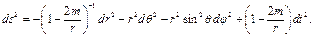Interior solution: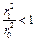i.e.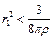and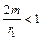i.e.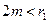Schwarzschild developed exterior solution adding tensors of empty space, cosmological constant, spherical polar coordinate and so on. He also added a new dimension as ‘r’ in his two solutions which is known as ‘Schwarzschild radius’ (Biswas, 2007) that means r = o which expresses zero tensor. Schwarzschild developed exterior solution from linear to spherical where 2m/r represents the boundary of the isolated particle whose radius must be greater than 2m (Prakash, 1985) and dr2 + r2dq2 + r2sin2qdf2 = polar coordinate, 2m/r where ‘r’ means radius and 2m means boundary but 2m <1. In empty space how can r control 2m without the force of gravitation? Thus it is an absurd idea. Whatsoever, Schwarzschild develops spherical coordinates in his exterior solution adding ‘dt’ as fourth dimension that creates spherical motion and measures the advance of perihelion of mercury. Schwarzschild also shows in interior solution that (1 >2m/r & 2m< r). Here the concept (1>2m/r) is taken from special relativity and it is a Newtonian idea. If (1 >2m/r), how (2m/r) controls the universe. Unification theory applies (r> 2m>1) where unified force controls the universe more speed of light. Here 1=motion of light, r= radius and m= mass.

14. Mathematical Analysis

14.1. Spherical Geometry

Here it is not possible to discuss the whole gamut of mathematics but to know how to change the linear geometry to spherical geometry. As discussed earlier that spherical geometry is necessary. Actually all dimensional geometry is included in spherical geometry. Geometry describes hundreds of laws of nature. Thus spherical geometry in the form of unification theory describes all the laws of the universe. Riemann successively developed three dimensional geometry and also spherical geometry. But it’s a matter of regret that the prospect of Riemann was not understood by mathematicians of his age and even by present age. Contemporary mathematician comments about Riemann that ‘Riemannian curvature is equal to the Gaussian curvature’ (Synge and Schild, 1969). It is miserable. N-dimensions of geometry have immense possibility. When fixed numbers are added with each Cartesian co-ordinates (x, y, z) and it becomes N-dimension co-ordinates. When time is added with spherical co-ordinates, it gets spherical motion. This spherical motion stands to single dimension. This metric is known as line element which is used in spherical geometry. Hawking says, ‘Thanks to Riemann’s investigation of N-dimensional metrical spaces’ (Hawking, 2007). Riemann drew line element from Pythagorean law of right angled triangle where (hypotenuse)2 is equal to (base)2 + (perpendicular)2. Example of three dimensional line element, ds2 = dx2 +dy2 +dz2. Adding time with N- dimension stands to single dimension is shown here as (i, j, k=1,2,3,4 ) in Figure 2.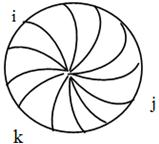Figure 2. N-dimensions stand to single dimension.

Spherical geometry of single dimension may be developed in four ways. Riemann transformed three dimensional Cartesian co-ordinates (x, y, z) into two types of spherical co-ordinates. One is N-dimensional metrical coordinates and the other is polar spherical co-ordinates (r, q, f).

Examples:

1) N-dimensional spherical tensor: ds2 = gijk dxi dxj dxk ( i , j, k = 1,2,3)

2) Polar spherical tensor: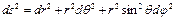3) Einstein developed tensor of four dimensions joining one of time with three of space. Perhaps Einstein did it without his knowing mind because he was not aware of that if time is added with space-time, it would revolve. His tensor was also defective as he used zero tensor in his metric (vide: Einstein’s law of gravitation).

Example: Einstein’s four dimensional metric: ds2= -dx2-dy2 –dz2+c2 dt2

4) The first author claimed to develop spherical tensor of single dimension as line element for unified force. Here gravitation interacts upon three basic forces lying in the inertia of mass of the earth and unified force is formed and it works cyclic way. It has supremacy over three spherical coordinates. Here r means radius and 2pr means boundary of the unified force (vide: Equation of unified force).

Example, Line element of unified law: ds2µ 2pr.

Finally each geometry merges with spherical geometry that helps to build unification theory that describes all physical laws of the universe.

14.2. Spherical Motion of Gravitation

As mentioned earlier unified force is related to gravitation. Gravitation works in spherical way like water cycle, air cycle, electromagnetic cycle etc. Without gravitational spherical movement planets would not move and universe will not work. It creates gravitational energy in high space controlling the planets. We get energy from electron so it is the case of gravitational particle. Spacecraft may work in high space depending upon gravitational energy. It has same spherical equation as that of Maxwell’s equations. Here fifth equation is added with four equations of Maxwell. But the formula of the equation would be spherical. The equations are as follows:

First equation: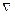.E ═ ρ/є0

Second equation:. B═ 0

Third equation:× E + B / t ═ 0

Fourth equation:×B- є0μ0 E/ t ═ μJ

Fifth equation:× ds2 µ 2pr

Here, J is the current density and ρ is the charge density. The ∂ is analogous in some ways to the d and ∂B/∂t is called the partial derivatives of B with respect to t. The equation.E is called the divergence of E and× E is the curl of E.× ds2 is the cyclic order of the electric field. Nablais the operator.

The significance of Maxwell’s equation is as follows: The first equation is Gauss’s law which follows from Coulomb’s law. It says that electrical lines of force begins or ends on electrical charges (ρ). The second equation, which is analogous to the first, contains the experimental fact that there are no magnetic charges; consequently, magnetic lines of force have no beginning or ending, but instead form closed loops. The third equation is Faraday’s law of induction, equivalent to the flux rule. A changing magnetic flux produces an electric field. The fourth equation states, analogously, that a changing flux of electrical lines of force produces a magnetic field, and also that a flow of electrical charges ( J ) produces a magnetic field (Good, 1974) and they work in cyclic way. The fifth equation over all proves that magnetic field propagates in cyclic way all over the world.

14.3. Structure of Unified Force

At first, four basic forces of quantum mechanics form unified force which may be called four in one or force of single dimension and simultaneously four properties of matter such as radius of circle, mass of the body, cyclic frequency and time join the unified force and make it workable. Thus five forces make unified force.

14.4. Equation of Unified Force

Unified force is used in two ways in two names such as in the form of gravitational attraction in high space and in the form of inertial force in the surface of the earth and air. The planets are attracted by unified force and vehicles are drawn by inertial force. Unified force itself is a spherical force. Unified equation of five forces makes line element. It has somewhat similarity with exterior solution of Schwarzschild. Comparison of exterior solution with unified law is shown as (dr2+r2dq2+ r2sin2 qdf2 = 2p r means boundary of the unified force, r means radius that controls the boundary and t = time).

Example of unified law: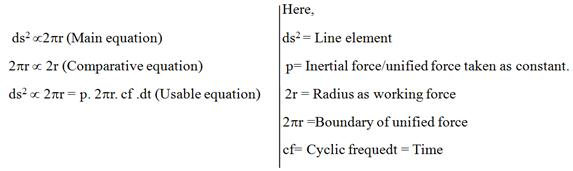14.5. Meaning of Unified Force

The idea of equation is taken from Radian of Trigonometry where circumference = 2pr (circumference /diameter =p, or, circumference = pd = 2pr). Here the concept 2pr represents the boundary of the unified force whose radius ‘r’ controls the boundary. In the formula, 2pr means unified force and ‘r’ means radius that controls unified force spherically. Inertial and gravitational mass both are considered as constant because inertia derives from a gravitation which is a constant force. Example: how five forces work united shown in figure 3.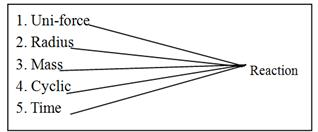Figure 3. Reaction of five properties of matter.

14.6. Comparison of Unified Equation

In comparative equation, motion in gravitational field is compared with that of non-gravitational field. Here a motion in gravitational field moves 2pr (circumference) in a circle within a fixed time, that motion in non-gravitational field moves equivalent to 2r (diameter) of that circle. The motion is linear and uniform in non-gravitational field but that of in gravitational field is spherical and accelerated. Newtonian laws of motion are linear and uniform but unified motion is spherical and accelerated. This comparison is shown in Figure 4.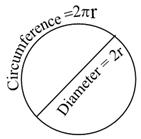Figure 4. Comparison between linear and spherical motion in a circle.

14.7. Explanation of Unified Forces

At first four basic forces form unified force through interaction and at the same time four properties of matter such as radius of the circle, mass of the body, cyclic force and time join unified force. Here reaction is greater than action because unified force works in cyclic way. Suppose a vehicle on earth and a planet in high space use unified force respectively.

14.8. Principle of Unified Force

Unification theory has its own philosophy. In gravitational field all bodies remain in the form of balance or round shape such as stars, planets, globe etc. As a result body moves by less force rather than classical force. This is the way buses, trains and vehicles move by less force on wheels. Whatever force is applied here, it would move the body once and extra force would supply by cyclic frequency. Thus the applied force would be considered as constant. The logic is that inertia mass derives from gravitational mass if gravitational force is constant, inertia force would be constant. Schwarzschild in his exterior solution exhibits mass and gravitation both as constant. If more force is applied more advantages will not be added with reaction because the cyclic frequency is there for supplying additional force. Gravitation influences inertia mass on the earth in two ways that is, gravitation static body stands to mass and moving body stands to acceleration (Tajuddin et al., 2007 and 2008). How a round body moves easily in a cyclic way is shown in Figure 5.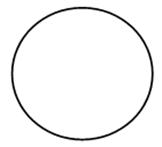Figure 5. A round body moves easily in cyclic way.

In classical physics of non-gravitational field, one body acts upon other in the form of proportion of their masses. Suppose, a body of 40 grams acts upon another body of 10 grams, the reaction would be 4 cm/ second2.

14.9. Four Properties of Matter

14.9.1 Mass of the Body

Mass of the body is a factor. In physics it is known as applied force. Massive body The planets move around the sun in sequence of distance due to their density requires more force. of mass. It goes with the Law of inertia by Newton.

A straight line from centre to any point on circumference in a circle is known as radius or Schwarzschild radius. Schwarzschild introduces the idea of radius in his solution. Radius controls unified force. Importance of radius is shown in the formula ( ds2µ 2pr) of unified force.

14.9.3. Cyclic Frequency

Unified force moves the body spherical way. A vehicle rotates on wheel several times before resuming full motion which is known as cyclic motion or spherical motion. After cyclic motion body gets uniform motion. Suppose, a vehicle moving 8 times gets full motion. This 8 time movements are known as cyclic motion. Mathematical analysis would be 2prÍ 8 = 16pr.

14.9.4. Time

Motion is always counted in respect of time. Time is always involved with motion. Time is counted as how many times. Here t is used as time (Tajuddin, 2014 ).

15. Useable Equation

Example: 40 dine of force acts 8 seconds upon 10 gm of body, how much distance the second body will move? (According to unified force all bodies are round shape or balanced. Suppose: bus, train, vehicle etc. move on wheels that has radius, cyclic frequency etc. Here the radius of the body is 2 cm. and starting period (cyclic frequency) 4 times).

Solution:

According to unified law

ds2 µ p .2pr .cf . t

= inertial force. unified force. radius. cyclic frequency & time

= 1 . 2pr .4 . 8

= 1.2. 22/7.2 .4. 8

= 201.15 cm

Here

P = inertia force /gravitational force.

2pr= boundary of unified of force.

c f = cyclic frequency (4 time)

t = time (8 seconds)

ds2  = ?

According to unified force, body will go 201.15 cm. and in classical mechanics it will go 128 cm only. (s= ut + ½ft2 = 0.8 + ½.4. (8)2 =128 cm.).

16. Conclusions

Unification theory has immense importance. Basic forces form unified force through interaction among themselves and properties of matter make it more workable. It has become the fifth basic force and the most powerful force that controls the universe. The great sayings of Hawking regarding unification theory is ‘a complete unified theory that will describe every thing in the universe’ have been proved. This force has some innovative ideas and techniques that have been discussed here.

Acknowledgements

Authors are grateful to late Prof. Jamal Nazrul Islam, RCMPS, Chittagong University, Bangladesh and Prof. Nurul Islam of Dhaka University, Bangladesh and others who helped them developing this article.

References

1. Biswas, M. H. A. (2010). "Mathematics and Physics of Collapsing Stars", ISBN: 978-3-6392-2132-9, VDM Verlag, Germany.
2. Biswas, M. H. A. (2007). Black Holes: The Ultimate Fate of the Collapsing Stars. Khulna University Studies, 8(1): 143—150.
3. Biswas, M. H. A.,Azad, M. A. K. and Ansary, M. A. (2009). Calculation of Mass Energy Density for a Collapsing Dust Sphere. Malaysian Journal of Mathematical Sciences, 3(2): 265—277.
4. Biswas, M. H. A.and Haque, T. F. (2009). The Relativistic Acceleration Relations Based on Force Transformation. Ozean Journal of Applied Sciences, 2(2): 229—239.
5. Biswas, M. H. A.,Jahan, S. S., Asaduzzaman, C. M., Kamruzzaman, M. and Rahman, M. A. (2011). Solution and Singularity Analysis of Einstein’s Equation to the Gravitational Field of Mass Point. Mathematical Forum, Vol. XXIII, 2010—2011, September 2011.
6. Biswas, M. H. A., Jahan, S. S., Asaduzzaman, C. M. and Kamruzzaman, M. (2008a). Exact solution of Einstein’s equation to the gravitational field of mass point in polar coordinates and the singularity analysis of the solution. Ozean Journal of Applied Sciences, 1(1): 29—38.
7. Biswas, M. H. A.,Mollick, U. K., Pervin, S. and Islam, A. (2008b). Curvature Invariants of Spherically Symmetric Schwarzschild Solution without Cosmological Constant. Journal of Applied Sciences Research, 4(1): 16—31.
8. Born, M. (1962). "Einstein’s Theory of Relativity", Dover Publications Inc., New York.
9. Calder, N. (1978). "Einstein’s Universe", Penguin Group, 27 Wright Lane, London, England, UK.
10. Fauvel, J. et. al. (1988). "Let Newton Be", Oxford University Press, U. K.
11. Good, R.H. (1974). "Basic Concepts of Relativity", East-West Edition, New Delhi, India.
12. Goldstein, A. M. (1999). "A Dictionary of Physics", CBS Publishers & Distributors, New Delhi, India.
13. Haque, T. F. and Biswas, M. H. A. (2010). "Relativistic Acceleration Relations Based on Force Transformations", ISBN:978-3-8383-4957-2, Lambert Academic Publishing, Germany.
14. Hawking, S.W. (1996). "A Brief History of Time", Bantam Books, New York.
15. Hawking, S. W. (2007). "The Essential Einstein: His Greatest Works", Penguin Books, London, U.K.
16. Hawking, S. W. (1993). "Black Holes And Baby Universes and Other Essays", Bantam Books, New York.
17. Prakash, S. (1990). "Relativistic Mechanics", Pragati Prakashan, Meerut, India.
18. Strathern, P. (1997). "Einstein and Relativity", Arrow Books, UK.
19. Synge, J. L. and Schild, A. (1978). "Tensor Calculus", p. 96, Dover Publications Inc. New York, 1978.
20. Tajuddin, M. (2014). "Einstein: Unification of Basic Forces" (in Bengali), Mau Publication, Dhaka, Bangladesh.
21. Tajuddin, Biswas, M. H. A. and Uddin, M. S. (2007). New Aspects of Principle of Equivalence. Acta Ciencia Indica, Vol. XXXIV M, No. 4, pp. 1931—1939.
22. Tajuddin, Biswas, M. H. A.and Islam, A. (2008). Review of the Theory of Relativity. Journal of Applied Sciences Research, 4(1): 32—39.
23. Tajuddin and Biswas, M. H. A. (2005). Unification of the Theory of Albert Einstein by Cyclic Theory, Asian J. Inform. Tech., 4(10): 962-970.
24. White, M. and Gribben, J. (2005). "A Life of Science: Einstein", p. 249, The Free Press, London, UK.

 Contents 1. 2. 3. 4. 5. 6. 7. 8. 9. 10. 11. 12. 13. 14. 14.1. 14.2. 14.3. 14.4. 14.5. 14.6. 14.7. 14.8. 14.9. 15. 16.
Article ToolsAbstractPDF(290K)